Plot matalbThe Plot Function

The plot function usually takes two arguments (but can take one). The first is the X values of the points to plot, and the second is the Y value of the points to plot. Below is an example of creating and plotting the values of the X squared graph from -10 to +10. In the case of a single argument, the X axis becomes 1,2,3,4,... up to the length of the array, and the Y axis contains the values of the array.

Notice that plot will "connect the dots" for you, drawing lines between each set of X,Y values.

If you have a single array that you wish to plot, and don't really care about the X axis, you just want to see the values plotted in the Y axis, you can pass a single array to the plot function. Here is an example:

The following functions are almost always used with plot to make the output more readable.

1. xlabel, ylabel : Provide a labeling for the graph axii.
2. title : Provide a title for the graph.
3. legend: Provide a legend telling what multiple graph lines mean.
4. grid: Put a checkered grid over the graph (add more lines to (sometimes) make it easier to see values.
5. axis: Tell how big of an area the graph should display.

Sometimes you will want to "clear" the figure. This is normally done by simply "plotting" the next thing you want. Alternatively, you can use the "clf" command:

Sometimes you will want to plot multiple graphs on the XY axis (on the same figure). In Matlab, when you use the plot function more than once, each time you call it, the previous figure is "erased".

To make a previous figure remain on the plot, we use the "hold on;" command. When we want to resume clearing the figure for each new plot, we use the "hold off;" command.

Try the following code:

You should have only seen one graph, the cubed graph (which was the second plot above). Now try this code, using the "hold on;" command.

Sometimes you will want to place multiple plots side by side on a single figure. You can achieve this by using the Matlab subplot function.

For many more details on this function (as well as the commands and functions mentioned above) you can always use the help command at the Matlab prompt.

The parameters used by the subplot function determine how many rows and columns for the overall "matrix" of figures, and then which of these positions to put the next plot in. The parameters are: 1) The number of rows, 2) the number of columns, 3) which of the sub plots to use.

Here is an example of how to create a 2x3 matrix (6 figures) of plots and to address each one of them by a number (shown below). Notice how row 1, column 1, is identified by the number 1. Row one, column three is identified by 3. Row two, column one, "wraps" and is identified by 4.:

Columns
onetwothree
Rowsone123
two456

Test this code. Notice that the subplot command stays the same, except for the "index" into which matrix location you want. Also notice that you can title, label, etc, each plot.

Matlab provides several well known graphing functions, including histograms, pie charts, and stem charts.

1. Histograms: Histograms are used to show how many values in an array of values fall within certain "bins". For example, if we have the scores of all students on a test, we may want to find out how many scores were between 90-100, 80-90, 70-80, etc. The histogram is the perfect tool for this.

2. Pie Charts: Pie Charts are useful for showing what percentage of a whole, each element represents. For example, if we knew there were 40 hours in the week, we could use a pie chart to show how many hours were spent on study, sleep, play, etc

3. Stem Charts: The stem chart draws a line from the bottom of the graph to the appropriate Y value for each X value. For example if the X axis represents how many times a letter was found in a document, we could use a stem plot to show the relationship between all letters, and how often they occur..

Sours: https://www.cs.utah.edu/~germain/PPS/Topics/Matlab/plot.html

Select a Web Site

Specify Line and Marker Appearance in Plots

MATLAB® creates plots using a default set of line styles, colors, and markers. These defaults provide a clean and consistent look across the different plots you create. If you want, you can customize these aspects of your plot. Many plotting functions have an input argument called for customizing. Also, the objects returned by these functions typically have properties for controlling these aspects of your plot. The names of the arguments and properties can vary, but the values they accept typically follow a common pattern. Once you are familiar with the pattern, you can use it to modify a wide variety of plots.

The following examples use the function to demonstrate the overall approach for customizing the appearance of lines. For a complete list of options for a specific plotting function, refer to the documentation for that function.

Line Styles

Most line plots display a solid line by default, but you can customize the line with any of the line styles in the following table. For example, create a line plot with a dashed line:

Line StyleDescriptionResulting Line
Solid line
Dashed line
Dotted line
Dash-dotted line

Markers

Usually, you can specify a marker symbol in addition to the line style. The markers appear at the data points in your chart. For example, create a line plot with a dashed line and circular markers:

MarkerDescriptionResulting Marker
Circle
Plus sign
Asterisk
Point
Cross
Horizontal line
Vertical line
Square
Diamond
Upward-pointing triangle
Downward-pointing triangle
Right-pointing triangle
Left-pointing triangle
Pentagram
Hexagram

Specify Combinations of Colors, Line Styles, and Markers

Many plotting functions have a single argument for specifying the color, the line style, and the marker. For example, the function has an optional argument for specifying one or more of these aspects. (Alternatively, you can set properties to modify the plot after creating it.)

Create a plot with a red dashed line and circular markers by specifying the argument as . For this combination, corresponds to a dashed line, corresponds to circular markers, and corresponds to red.

plot([1 2 3 4 5 6],[0 3 1 6 4 10],'--or')

You do not need to specify all three aspects of the line. For example, if you specify only the marker, the plot displays the markers with the default color and no line.

plot([1 2 3 4 5 6],[0 3 1 6 4 10],'o')

You can use the argument to specify a named color, but to specify a custom color, set an object property. For example, objects have a property.

Create a plot with a purple line that has circular markers. Specify only the line and marker symbols in the argument. Set the property separately as a name-value argument. Return the object as , so you can change other properties later.

p = plot([1 2 3 4 5 6],[0 3 1 6 4 10],'-o','Color',[0.5 0 0.8]);

Next, change the color of the line to a shade of green by setting the property to the hexadecimal color code . Then change the line style to dashed, and change the markers to asterisks.

Before R2019a, specify the color as an RGB triplet instead of a hexadecimal color code. For example, .

p.Color = '#00841a'; p.LineStyle = '--'; p.Marker = '*';

Modify Line Width, Marker Fill, and Marker Outline

You can modify other aspects of lines by setting properties. For example, objects have a property for controlling the line's thickness. To create a thicker line, you can specify the as a name-value argument when you call the function. In this case, set the to . Return the object as so you can set other properties later.

p = plot([1 2 3 4 5 6],[0 3 1 6 4 10],'-o','LineWidth',3);

Fill the markers with a shade of orange by setting the property on the object. Then increase the marker size to by setting the property.

p.MarkerFaceColor = [1 0.5 0]; p.MarkerSize = 8;

Change the outlines of the markers to match the fill color by setting the property.

p.MarkerEdgeColor = [1 0.5 0];

Related Topics

Sours: https://www.mathworks.com/help/matlab/creating_plots/specify-line-and-marker-appearance-in-plots.html

MATLAB

In this section we will use MATLAB ’s plot command to produce graphs. In Sections 6 and 8 you will see there are two other commands to create graphs, namely fplot which uses function M-files and ezplot which is inside the Symbolic Math Toolbox.

If x = {x(1), x(2), . . . , x(n )} and y = {y(1), y(2), . . ., y(n)}, then the MATLAB command plot(x,y) opens a graphics window, called a Figure window, scales the axes to fit the data, plots the points (x(1), y(1)), (x(2), y(2)), . . . , (x(n), y(n)), and then graphs the data by connecting the points with straight lines. If a Figure window already exists, another plot command clears the current Figure window by default (unless instructed not to do so) and draws a new plot. Type in the following as an attempt to graph y = sinx for 0 ≤ x ≤ 2π. [You could replace the last two lines with plot(x,sin(x)).]

clear all

x=linspace(0,2*pi,11);

y=sin(x);

plot(x,y)

Clearly this attempt is unacceptable because we have not used sufficient points! Change the 11 to 201 and execute the code again.

In general, most graphs can be satisfactorily plotted using arrays of, say, 101 to 201 points, although erratic or wildly fluctuating functions would require many more points. Please note that you only need two points to plot a single straight line. For example, to plot a straight line from the point (1,7) to the point (3,-5) you need the command plot([1 3],[7 -5]).

Note: Students often get this wrong by forgetting that the first array always contains the x-coordinates, not the two coordinates of the first point. Similarly, the second array contains the y-coordinates.

Exercise 1: Plot the graph of y = xex/x2 − π2 for −3 ≤ x ≤ 2 using a step-size of 0.02. You will need three dots in the expression to generate the array y.

Exercise 2: Plot the graph of y = sin9x + sin10.5x + sin12x for −π ≤ x ≤ π using 601 points.

Plotting several graphs on the same axes

Example 1: Suppose you want to plot the oscillations y1 = cost, y2 = cos3t and their sum y3 = y1 + y2, for 0 ≤ t ≤ 4π, on the same axes. Here t is measured in seconds and y1, y2, y3 are measured in cm.

clear all

t=linspace(0,4*pi,201);

y1=cos(t); y2=cos(3*t); y3=y1+y2;

plot(t,y1,t,y2,t,y3)

You notice that we found the three y-arrays in a single line of code and they were plotted in different colours. But the empty space at the right end of the graphs is annoying, and how can we label the output so that each graph can be identified? There are many facilities provided by MATLAB to assist you in producing attractive, meaningful graphs. Change the above M-file to the following (this is important!):

clear all

t=linspace(0,4*pi,201);

y1=cos(t); y2=cos(3*t); y3=y1+y2;

axis([0 4*pi -2 2]) % specifies the axes limits

hold on

plot(t,y1,’b--’,t,y2,’g:’,t,y3,’r’)

legend(’y1=cos(t)’,’y2=cos(3*t)’,’y3=y1+y2’)

plot([0 4*pi],[0 0],’k’) % adds the t axis in black

xlabel(’time in seconds’)

ylabel(’displacement in cm’)

title(’oscillations’)

hold off

A short diversion

To get assistance with the MATLAB commands featured above and in the next example, you can use the help facility. Enter each of the following after the >> prompt and carefully read the information until you understand precisely what each of the lines in the previous (or next) M-file is accomplishing.

• help axis
• help plot
• help hold
• help legend
• help text

Alternatively, if you do not know the precise name of a MATLAB command for which you need help, you can click on the Help button at the top of the MATAB Command Window, then click on “MATLAB Help” followed by “MATLAB Functions Listed by Category” and then on a topic of interest. For example, to get help on many graphics related commands, click on Plotting and Data Visualization.

Another help option is the lookfor facility. Suppose you were interested in commands involving the use of complex numbers. After the >> prompt enter lookfor complex.

Example 2: By using hold on and hold off, you can plot several functions on the same axes using a number of plot commands. The functions f(x) = x2 and f−1(x) = √x are inverse functions for x ≥ 0 and hence their graphs must be reflections through the line y = x. Execute the following M-file, carefully noting the results of each command:

clear all % file available in M-files folder as file1.m

axis([0 4 0 4]), axis square

hold on

x1=linspace(0,2,101);

y1=x1.^2; % note the dot

plot(x1,y1)

x2=linspace(0,4,101);

y2=sqrt(x2);

plot(x2,y2)

plot([0 4],[0 4],’k:’) % dotted line in black from (0,0) to (4,4)

text(1.5,3.5,’y=x^2’)

text(3.1,1.7,’y=sqrt(x)’)

grid on % adds grid lines if you want them

title(’reflection property of inverse functions’)

Zoom on

MATLAB provides an interactive tool to expand sections of a plot to see more detail. This is particularly useful if you need to obtain accurate information about where two graphs intersect, or to find the coordinates of an extreme point. The command zoom on, either in your M-file or after the >> prompt turns on the zoom mode. Then go to the Figure window, place the pointer where you want an enlargement and click with the left button. Continue this process and you can zoom in to obtain three or four decimal place accuracy.

Example: Suppose you want to find graphically the point of intersection of y = tanx and y = 1− x3. (Note that there are other non-graphical ways of doing this, described in Sections 6 and 8.) Firstly, plot both of these functions on the same axes for −1.2 ≤ x ≤ 1.2, with at least 800 points to enable zooming. You might also like to add the x and y axes to obtain a figure similar to that on the previous page. Enter the command zoom on. Then zoom in at the point of intersection until you are able to find its coordinates to the accuracy of (0.6376 , 0.7408).

Exercise: Plot y = 10e−t sin t for 0 ≤ t ≤ 2π and then use zoom on to find its maximum value.

Creating separate graphs in one M-file

If you want two separate Figures to be created in one M-file, you can use the figure(n) command where n is a number associated with the window and precedes the set of plotting

commands.

Example: At time t seconds, t ≥ 0, a moving point has coordinates x = sin2t, y = cos3t (metres), and so its speed is given by

Plot the path taken by the point over one cycle 0 ≤ t ≤ 2π, where t is regarded as a parameter, and also plot the speed against time. Use the following M-file. (You can add your own labels, etc.)

clear all % file available in M-files folder as file2.m

t=linspace(0,2*pi,500);

x=sin(2*t);

y=cos(3*t);

figure(1)

plot(x,y)

axis equal % same scale (metres) on each axis

speed=sqrt(4*cos(2*t).^2+9*sin(3*t).^2); % note the dots

figure(2)

axis([0 2*pi 0 4])

hold on

plot(t,speed)

hold off

Sours: https://lo.unisa.edu.au/mod/book/view.php?id=466690&chapterid=75030
3D Plotting in Matlab

MATLAB - Plotting

To plot the graph of a function, you need to take the following steps −

• Define x, by specifying the range of values for the variable x, for which the function is to be plotted

• Define the function, y = f(x)

• Call the plot command, as plot(x, y)

Following example would demonstrate the concept. Let us plot the simple function y = x for the range of values for x from 0 to 100, with an increment of 5.

Create a script file and type the following code −

x = [0:5:100]; y = x; plot(x, y)

When you run the file, MATLAB displays the following plot −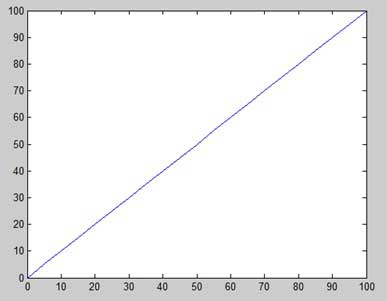Let us take one more example to plot the function y = x2. In this example, we will draw two graphs with the same function, but in second time, we will reduce the value of increment. Please note that as we decrease the increment, the graph becomes smoother.

Create a script file and type the following code −

x = [1 2 3 4 5 6 7 8 9 10]; x = [-100:20:100]; y = x.^2; plot(x, y)

When you run the file, MATLAB displays the following plot −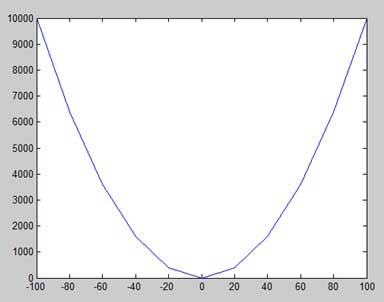Change the code file a little, reduce the increment to 5 −

x = [-100:5:100]; y = x.^2; plot(x, y)

MATLAB draws a smoother graph −Adding Title, Labels, Grid Lines and Scaling on the Graph

MATLAB allows you to add title, labels along the x-axis and y-axis, grid lines and also to adjust the axes to spruce up the graph.

• The xlabel and ylabel commands generate labels along x-axis and y-axis.

• The title command allows you to put a title on the graph.

• The grid on command allows you to put the grid lines on the graph.

• The axis equal command allows generating the plot with the same scale factors and the spaces on both axes.

• The axis square command generates a square plot.

Example

Create a script file and type the following code −

x = [0:0.01:10]; y = sin(x); plot(x, y), xlabel('x'), ylabel('Sin(x)'), title('Sin(x) Graph'), grid on, axis equal

MATLAB generates the following graph −Drawing Multiple Functions on the Same Graph

You can draw multiple graphs on the same plot. The following example demonstrates the concept −

Example

Create a script file and type the following code −

x = [0 : 0.01: 10]; y = sin(x); g = cos(x); plot(x, y, x, g, '.-'), legend('Sin(x)', 'Cos(x)')

MATLAB generates the following graph −Setting Colors on Graph

MATLAB provides eight basic color options for drawing graphs. The following table shows the colors and their codes −

CodeColor
wWhite
kBlack
bBlue
rRed
cCyan
gGreen
mMagenta
yYellow

Example

Let us draw the graph of two polynomials

• f(x) = 3x4 + 2x3+ 7x2 + 2x + 9 and

• g(x) = 5x3 + 9x + 2

Create a script file and type the following code −

x = [-10 : 0.01: 10]; y = 3*x.^4 + 2 * x.^3 + 7 * x.^2 + 2 * x + 9; g = 5 * x.^3 + 9 * x + 2; plot(x, y, 'r', x, g, 'g')

When you run the file, MATLAB generates the following graph −Setting Axis Scales

The axis command allows you to set the axis scales. You can provide minimum and maximum values for x and y axes using the axis command in the following way −

axis ( [xmin xmax ymin ymax] )

The following example shows this −

Example

Create a script file and type the following code −

x = [0 : 0.01: 10]; y = exp(-x).* sin(2*x + 3); plot(x, y), axis([0 10 -1 1])

When you run the file, MATLAB generates the following graph −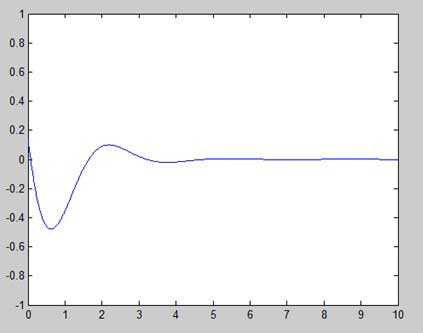Generating Sub-Plots

When you create an array of plots in the same figure, each of these plots is called a subplot. The subplot command is used for creating subplots.

Syntax for the command is −

subplot(m, n, p)

where, m and n are the number of rows and columns of the plot array and p specifies where to put a particular plot.

Each plot created with the subplot command can have its own characteristics. Following example demonstrates the concept −

Example

Let us generate two plots −

y = e−1.5xsin(10x)

y = e−2xsin(10x)

Create a script file and type the following code −

x = [0:0.01:5]; y = exp(-1.5*x).*sin(10*x); subplot(1,2,1) plot(x,y), xlabel('x'),ylabel('exp(–1.5x)*sin(10x)'),axis([0 5 -1 1]) y = exp(-2*x).*sin(10*x); subplot(1,2,2) plot(x,y),xlabel('x'),ylabel('exp(–2x)*sin(10x)'),axis([0 5 -1 1])

When you run the file, MATLAB generates the following graph −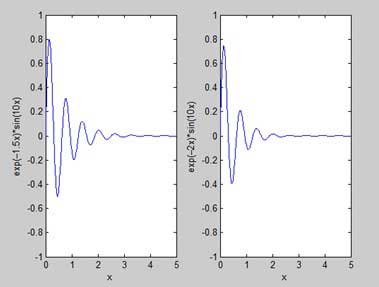Sours: https://www.tutorialspoint.com/matlab/matlab_plotting.htm

Matalb plot

 MATLAB Function Referenceplot

Linear 2-D plot

Syntax

• plot(Y) plot(X1,Y1,...) plot(X1,Y1,,...) plot(...,'',PropertyValue,...) plot(axes_handle,...) h = plot(...) hlines = plot('v6',...)

Description

plots the columns of versus their index if is a real number. If is complex, is equivalent to . In all other uses of , the imaginary component is ignored.

plots all lines defined by versus pairs. If only or is a matrix, the vector is plotted versus the rows or columns of the matrix, depending on whether the vector's row or column dimension matches the matrix.

plots all lines defined by the triples, where is a line specification that determines line type, marker symbol, and color of the plotted lines. You can mix triples with pairs: .

 Note    See for a list of line style, marker, and color specifiers.

sets properties to the specified property values for all lineseries graphics objects created by . (See the "Examples" section for examples.)

plots into the axes with handle axes_handle instead of the current axes ().

returns a column vector of handles to lineseries graphics objects, one handle per line.

Backward Compatible Version

returns the handles to line objects instead of lineseries objects.

Remarks

If you do not specify a color when plotting more than one line, automatically cycles through the colors in the order specified by the current axes property. After cycling through all the colors defined by , then cycles through the line styles defined in the axes property.

The default property has a single entry (a solid line with no marker).

Cycling Through Line Colors and Styles

By default, MATLAB resets the and properties each time you call . If you want changes you make to these properties to persist, then you must define these changes as default values. For example,

• set(0,'DefaultAxesColorOrder',[0 0 0],...       'DefaultAxesLineStyleOrder','-|-.|--|:')

sets the default to use only the color black and sets the to use solid, dash-dot, dash-dash, and dotted line styles.

Prevent Resetting of Color and Styles with hold all

The option to the command prevents the and from being reset in subsequent commands. In the following sequence of commands, MATLAB continues to cycle through the colors defined by the axes property (see above).

• plot(rand(12,2)) hold all plot(randn(12,2))

Examples

Specifying the Color and Size of Markers

You can also specify other line characteristics using graphics properties (see for a description of these properties):

• -- Specifies the width (in points) of the line.
• -- Specifies the color of the marker or the edge color for filled markers (circle, square, diamond, pentagram, hexagram, and the four triangles).
• -- Specifies the color of the face of filled markers.
• -- Specifies the size of the marker in units of points.

For example, these statements,

• x = -pi:pi/10:pi; y = tan(sin(x)) - sin(tan(x)); plot(x,y,'--rs','LineWidth',2,... 'MarkerEdgeColor','k',... 'MarkerFaceColor','g',... 'MarkerSize',10)

produce this graph.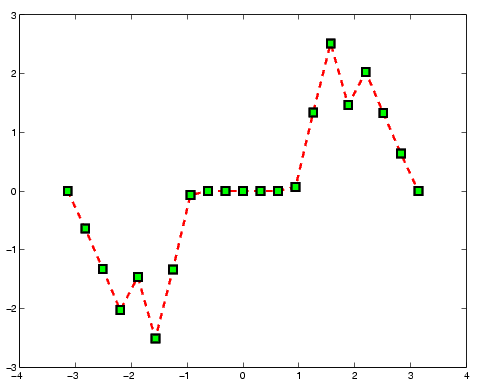Specifying Tick-Mark Location and Labeling

You can adjust the axis tick-mark locations and the labels appearing at each tick. For example, this plot of the sine function relabels the x-axis with more meaningful values:

• x = -pi:.1:pi; y = sin(x); plot(x,y) set(gca,'XTick',-pi:pi/2:pi) set(gca,'XTickLabel',{'-pi','-pi/2','0','pi/2','pi'})

Now add axis labels and annotate the point -pi/4, sin(-pi/4).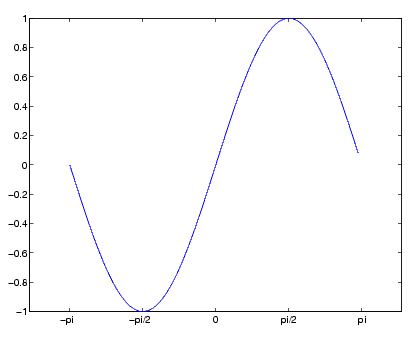Adding Titles, Axis Labels, and Annotations

MATLAB enables you to add axis labels and titles. For example, using the graph from the previous example, add an x- and y-axis label:

• xlabel('-\pi \leq \Theta \leq \pi') ylabel('sin(\Theta)') title('Plot of sin(\Theta)') text(-pi/4,sin(-pi/4),'\leftarrow sin(-\pi\div4)',...      'HorizontalAlignment','left')

Now change the line color to red by first finding the handle of the line object created by and then setting its property. In the same statement, set the property to 2 points.

• ((gca,'Type','line','Color',[0 0 1]),...     'Color','red',... 'LineWidth',2)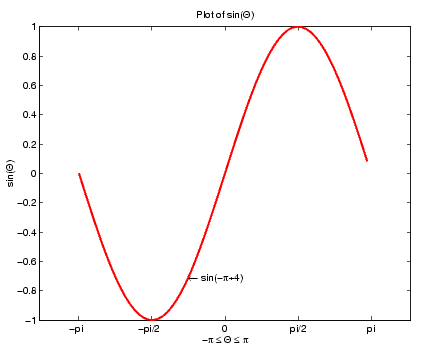, , , , , , , , , , , , , , , , , , , ,

See the property for a list of symbols and how to display them.

See the Plot Editor for information on plot annotation tools in the figure window toolbar.

See Basic Plots and Graphs for related functions.playshow plot3Sours: http://matlab.izmiran.ru/help/techdoc/ref/plot.html
Basic data plotting in MATLAB

plot

•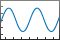Description

example

creates a 2-D line plot of the data in versus the corresponding values in .

• To plot a set of coordinates connected by line segments, specify and as vectors of the same length.

• To plot multiple sets of coordinates on the same set of axes, specify at least one of or as a matrix.

creates the plot using the specified line style, marker, and color.

example

plots multiple pairs of x- and y-coordinates on the same set of axes. Use this syntax as an alternative to specifying coordinates as matrices.

example

assigns specific line styles, markers, and colors to each x-y pair. You can specify for some x-y pairs and omit it for others. For example, specifies markers for the first x-y pair but not for the second pair.

example

plots against an implicit set of x-coordinates.

• If is a vector, the x-coordinates range from 1 to .

• If is a matrix, the plot contains one line for each column in . The x-coordinates range from 1 to the number of rows in .

If contains complex numbers, MATLAB® plots the imaginary part of versus the real part of . If you specify both and , the imaginary part is ignored.

specifies line style, marker, and color.

example

specifies properties using one or more name-value arguments. The properties apply to all the plotted lines. Specify the name-value arguments after all the arguments in any of the previous syntaxes. For a list of properties, see Line Properties.

example

displays the plot in the target axes. Specify the axes as the first argument in any of the previous syntaxes.

example

returns a object or an array of objects. Use to modify properties of the plot after creating it. For a list of properties, see Line Properties.

collapse all

Create Line Plot

Create as a vector of linearly spaced values between 0 and . Use an increment of between the values. Create as sine values of . Create a line plot of the data.

x = 0:pi/100:2*pi; y = sin(x); plot(x,y)

Plot Multiple Lines

Define as 100 linearly spaced values between and . Define and as sine and cosine values of . Create a line plot of both sets of data.

x = linspace(-2*pi,2*pi); y1 = sin(x); y2 = cos(x); figure plot(x,y1,x,y2)

Create Line Plot From Matrix

Define as the 4-by-4 matrix returned by the function.

Y = 4×4 16 2 3 13 5 11 10 8 9 7 6 12 4 14 15 1

Create a 2-D line plot of . MATLAB® plots each matrix column as a separate line.

Specify Line Style

Plot three sine curves with a small phase shift between each line. Use the default line style for the first line. Specify a dashed line style for the second line and a dotted line style for the third line.

x = 0:pi/100:2*pi; y1 = sin(x); y2 = sin(x-0.25); y3 = sin(x-0.5); figure plot(x,y1,x,y2,'--',x,y3,':')

MATLAB® cycles the line color through the default color order.

Specify Line Style, Color, and Marker

Plot three sine curves with a small phase shift between each line. Use a green line with no markers for the first sine curve. Use a blue dashed line with circle markers for the second sine curve. Use only cyan star markers for the third sine curve.

x = 0:pi/10:2*pi; y1 = sin(x); y2 = sin(x-0.25); y3 = sin(x-0.5); figure plot(x,y1,'g',x,y2,'b--o',x,y3,'c*')

Display Markers at Specific Data Points

Create a line plot and display markers at every fifth data point by specifying a marker symbol and setting the property as a name-value pair.

x = linspace(0,10); y = sin(x); plot(x,y,'-o','MarkerIndices',1:5:length(y))

Specify Line Width, Marker Size, and Marker Color

Create a line plot and use the option to specify a dashed green line with square markers. Use pairs to specify the line width, marker size, and marker colors. Set the marker edge color to blue and set the marker face color using an RGB color value.

x = -pi:pi/10:pi; y = tan(sin(x)) - sin(tan(x)); figure plot(x,y,'--gs',...'LineWidth',2,...'MarkerSize',10,...'MarkerEdgeColor','b',...'MarkerFaceColor',[0.5,0.5,0.5])

Use the function to define as a vector of 150 values between 0 and 10. Define as cosine values of .

x = linspace(0,10,150); y = cos(5*x);

Create a 2-D line plot of the cosine curve. Change the line color to a shade of blue-green using an RGB color value. Add a title and axis labels to the graph using the , , and functions.

figure plot(x,y,'Color',[0,0.7,0.9]) title('2-D Line Plot') xlabel('x') ylabel('cos(5x)')

Plot Durations and Specify Tick Format

Define as seven linearly spaced values between 0 and 3 minutes. Plot random data and specify the format of the tick marks using the name-value pair argument.

t = 0:seconds(30):minutes(3); y = rand(1,7); plot(t,y,'DurationTickFormat','mm:ss')

Specify Axes for Line Plot

Starting in R2019b, you can display a tiling of plots using the and functions. Call the function to create a 2-by-1 tiled chart layout. Call the function to create an axes object and return the object as . Create the top plot by passing to the function. Add a title and y-axis label to the plot by passing the axes to the and functions. Repeat the process to create the bottom plot.

% Create data and 2-by-1 tiled chart layout x = linspace(0,3); y1 = sin(5*x); y2 = sin(15*x); tiledlayout(2,1) % Top plot ax1 = nexttile; plot(ax1,x,y1) title(ax1,'Top Plot') ylabel(ax1,'sin(5x)') % Bottom plot ax2 = nexttile; plot(ax2,x,y2) title(ax2,'Bottom Plot') ylabel(ax2,'sin(15x)')

Modify Lines After Creation

Define as 100 linearly spaced values between and . Define and as sine and cosine values of . Create a line plot of both sets of data and return the two chart lines in .

x = linspace(-2*pi,2*pi); y1 = sin(x); y2 = cos(x); p = plot(x,y1,x,y2);

Change the line width of the first line to 2. Add star markers to the second line. Use dot notation to set properties.

p(1).LineWidth = 2; p(2).Marker = '*';

Plot Circle

Plot a circle centered at the point (4,3) with a radius equal to 2. Use to use equal data units along each coordinate direction.

r = 2; xc = 4; yc = 3; theta = linspace(0,2*pi); x = r*cos(theta) + xc; y = r*sin(theta) + yc; plot(x,y) axis equal

collapse all

— x-coordinatesscalar | vector | matrix

x-coordinates, specified as a scalar, vector, or matrix. The size and shape of depends on the shape of your data and the type of plot you want to create. This table describes the most common situations.

Type of PlotHow to Specify Coordinates
Single point

Specify and as scalars and include a marker. For example:

One set of points

Specify and as any combination of row or column vectors of the same length. For example:

Multiple sets of points
(using vectors)

Specify consecutive pairs of and vectors. For example:

plot([1 2 3],[4 5 6],[1 2 3],[7 8 9])
Multiple sets of points
(using matrices)

If all the sets share the same x- or y-coordinates, specify the shared coordinates as a vector and the other coordinates as a matrix. The length of the vector must match one of the dimensions of the matrix. For example:

plot([1 2 3],[4 5 6; 7 8 9])
If the matrix is square, MATLAB plots one line for each column in the matrix.

Alternatively, specify and as matrices of equal size. In this case, MATLAB plots each column of against the corresponding column of . For example:

plot([1 2 3; 4 5 6],[7 8 9; 10 11 12])

Data Types: | | | | | | | | | | | |

— y-coordinatesscalar | vector | matrix

y-coordinates, specified as a scalar, vector, or matrix. The size and shape of depends on the shape of your data and the type of plot you want to create. This table describes the most common situations.

Type of PlotHow to Specify Coordinates
Single point

Specify and as scalars and include a marker. For example:

One set of points

Specify and as any combination of row or column vectors of the same length. For example:

Alternatively, specify just the y-coordinates. For example:

Multiple sets of points
(using vectors)

Specify consecutive pairs of and vectors. For example:

plot([1 2 3],[4 5 6],[1 2 3],[7 8 9])
Multiple sets of points
(using matrices)

If all the sets share the same x- or y-coordinates, specify the shared coordinates as a vector and the other coordinates as a matrix. The length of the vector must match one of the dimensions of the matrix. For example:

plot([1 2 3],[4 5 6; 7 8 9])
If the matrix is square, MATLAB plots one line for each column in the matrix.

Alternatively, specify and as matrices of equal size. In this case, MATLAB plots each column of against the corresponding column of . For example:

plot([1 2 3; 4 5 6],[7 8 9; 10 11 12])

Data Types: | | | | | | | | | | | |

— Line style, marker, and colorcharacter vector | string

Line style, marker, and color, specified as a character vector or string containing symbols. The symbols can appear in any order. You do not need to specify all three characteristics (line style, marker, and color). For example, if you omit the line style and specify the marker, then the plot shows only the marker and no line.

Example: is a red dashed line with circle markers

Line StyleDescriptionResulting Line
Solid line
Dashed line
Dotted line
Dash-dotted line
MarkerDescriptionResulting Marker
Circle
Plus sign
Asterisk
Point
Cross
Horizontal line
Vertical line
Square
Diamond
Upward-pointing triangle
Downward-pointing triangle
Right-pointing triangle
Left-pointing triangle
Pentagram
Hexagram
Color NameShort NameRGB TripletAppearance

— Target axes object | object | object

Target axes, specified as an object, a object, or a object. If you do not specify the axes, MATLAB plots into the current axes or it creates an object if one does not exist.

To create a polar plot or geographic plot, specify as a or object. Alternatively, call the or function.

Name-Value Arguments

Specify optional comma-separated pairs of arguments. is the argument name and is the corresponding value. must appear inside quotes. You can specify several name and value pair arguments in any order as .

Example:

The chart line properties listed here are only a subset. For a complete list, see Line Properties.

— Line color (default) | RGB triplet | hexadecimal color code | | | | ...

Line color, specified as an RGB triplet, a hexadecimal color code, a color name, or a short name.

For a custom color, specify an RGB triplet or a hexadecimal color code.

• An RGB triplet is a three-element row vector whose elements specify the intensities of the red, green, and blue components of the color. The intensities must be in the range ; for example, .

• A hexadecimal color code is a character vector or a string scalar that starts with a hash symbol () followed by three or six hexadecimal digits, which can range from to . The values are not case sensitive. Thus, the color codes , , , and are equivalent.

Alternatively, you can specify some common colors by name. This table lists the named color options, the equivalent RGB triplets, and hexadecimal color codes.

Color NameShort NameRGB TripletHexadecimal Color CodeAppearance
Not applicableNot applicableNot applicableNo color

Here are the RGB triplets and hexadecimal color codes for the default colors MATLAB uses in many types of plots.

Example:

Example:

Example:

— Line style (default) | | | |

Line style, specified as one of the options listed in this table.

Line StyleDescriptionResulting Line
Solid line
Dashed line
Dotted line
Dash-dotted line
No lineNo line

— Line width (default) | positive value

Line width, specified as a positive value in points, where 1 point = 1/72 of an inch. If the line has markers, then the line width also affects the marker edges.

The line width cannot be thinner than the width of a pixel. If you set the line width to a value that is less than the width of a pixel on your system, the line displays as one pixel wide.

— Marker symbol (default) | | | | | ...

Marker symbol, specified as one of the values listed in this table. By default, the object does not display markers. Specifying a marker symbol adds markers at each data point or vertex.

MarkerDescriptionResulting Marker
Circle
Plus sign
Asterisk
Point
Cross
Horizontal line
Vertical line
Square
Diamond
Upward-pointing triangle
Downward-pointing triangle
Right-pointing triangle
Left-pointing triangle
Pentagram
Hexagram
No markersNot applicable

— Indices of data points at which to display markers (default) | vector of positive integers | scalar positive integer

Indices of data points at which to display markers, specified as a vector of positive integers. If you do not specify the indices, then MATLAB displays a marker at every data point.

Note

To see the markers, you must also specify a marker symbol.

Example: displays a circle marker at the first, fifth, and tenth data points.

Example: displays a cross marker every three data points.

Example: displays one square marker at the fifth data point.

— Marker outline color (default) | RGB triplet | hexadecimal color code | | | | ...

Marker outline color, specified as , an RGB triplet, a hexadecimal color code, a color name, or a short name. The default value of uses the same color as the property.

For a custom color, specify an RGB triplet or a hexadecimal color code.

• An RGB triplet is a three-element row vector whose elements specify the intensities of the red, green, and blue components of the color. The intensities must be in the range ; for example, .

• A hexadecimal color code is a character vector or a string scalar that starts with a hash symbol () followed by three or six hexadecimal digits, which can range from to . The values are not case sensitive. Thus, the color codes , , , and are equivalent.

Alternatively, you can specify some common colors by name. This table lists the named color options, the equivalent RGB triplets, and hexadecimal color codes.

Color NameShort NameRGB TripletHexadecimal Color CodeAppearance
Not applicableNot applicableNot applicableNo color

Here are the RGB triplets and hexadecimal color codes for the default colors MATLAB uses in many types of plots.

— Marker fill color (default) | | RGB triplet | hexadecimal color code | | | | ...

Marker fill color, specified as , an RGB triplet, a hexadecimal color code, a color name, or a short name. The option uses the same color as the property of the parent axes. If you specify and the axes plot box is invisible, the marker fill color is the color of the figure.

For a custom color, specify an RGB triplet or a hexadecimal color code.

• An RGB triplet is a three-element row vector whose elements specify the intensities of the red, green, and blue components of the color. The intensities must be in the range ; for example, .

• A hexadecimal color code is a character vector or a string scalar that starts with a hash symbol () followed by three or six hexadecimal digits, which can range from to . The values are not case sensitive. Thus, the color codes , , , and are equivalent.

Alternatively, you can specify some common colors by name. This table lists the named color options, the equivalent RGB triplets, and hexadecimal color codes.

Color NameShort NameRGB TripletHexadecimal Color CodeAppearance
Not applicableNot applicableNot applicableNo color

Here are the RGB triplets and hexadecimal color codes for the default colors MATLAB uses in many types of plots.

— Marker size (default) | positive value

Marker size, specified as a positive value in points, where 1 point = 1/72 of an inch.

— Format for tick labelscharacter vector | string

Format for tick labels, specified as the comma-separated pair consisting of and a character vector or string containing a date format. Use the letters and to construct a custom format. These letters correspond to the Unicode® Locale Data Markup Language (LDML) standard for dates. You can include non-ASCII letter characters such as a hyphen, space, or colon to separate the fields.

If you do not specify a value for , then automatically optimizes and updates the tick labels based on the axis limits.

Example: displays a date and time such as .

The following table shows several common display formats and examples of the formatted output for the date, Saturday, April 19, 2014 at 9:41:06 PM in New York City.

Value of Example

For a complete list of valid letter identifiers, see the property for datetime arrays.

is not a chart line property. You must set the tick format using the name-value pair argument when creating a plot. Alternatively, set the format using the and functions.

The property of the datetime ruler stores the format.

— Format for tick labelscharacter vector | string

Format for tick labels, specified as the comma-separated pair consisting of and a character vector or string containing a duration format.

If you do not specify a value for , then automatically optimizes and updates the tick labels based on the axis limits.

To display a duration as a single number that includes a fractional part, for example, 1.234 hours, specify one of the values in this table.

Value of Description
Number of exact fixed-length years. A fixed-length year is equal to 365.2425 days.
Number of exact fixed-length days. A fixed-length day is equal to 24 hours.
Number of hours
Number of minutes
Number of seconds

Example: displays duration values in terms of fixed-length days.

To display a duration in the form of a digital timer, specify one of these values.

In addition, you can display up to nine fractional second digits by appending up to nine characters.

Example: displays the milliseconds of a duration value to three digits.

is not a chart line property. You must set the tick format using the name-value pair argument when creating a plot. Alternatively, set the format using the and functions.

The property of the duration ruler stores the format.

Tips

• Use and values to create breaks in the lines. For example, this code plots the first two elements, skips the third element, and draws another line using the last two elements:

• uses colors and line styles based on the and properties of the axes. cycles through the colors with the first line style. Then, it cycles through the colors again with each additional line style.

Starting in R2019b, you can change the colors and the line styles after plotting by setting the or properties on the axes. You can also call the function to change the color order for all the axes in the figure.

Tall ArraysCalculate with arrays that have more rows than fit in memory.

Usage notes and limitations:

• Supported syntaxes for tall arrays and are:

• must be in monotonically increasing order.

• Categorical inputs are not supported.

• Tall inputs must be real column vectors.

• With tall arrays, the function plots in iterations, progressively adding to the plot as more data is read. During the updates, a progress indicator shows the proportion of data that has been plotted. Zooming and panning is supported during the updating process, before the plot is complete. To stop the update process, press the pause button in the progress indicator.

GPU ArraysAccelerate code by running on a graphics processing unit (GPU) using Parallel Computing Toolbox™.

Usage notes and limitations:

• This function accepts GPU arrays, but does not run on a GPU.

For more information, see Run MATLAB Functions on a GPU (Parallel Computing Toolbox).

Distributed ArraysPartition large arrays across the combined memory of your cluster using Parallel Computing Toolbox™.

Usage notes and limitations:

• This function operates on distributed arrays, but executes in the client MATLAB.

For more information, see Run MATLAB Functions with Distributed Arrays (Parallel Computing Toolbox).

Properties

Introduced before R2006a

You have a modified version of this example. Do you want to open this example with your edits?

You clicked a link that corresponds to this MATLAB command:

Run the command by entering it in the MATLAB Command Window. Web browsers do not support MATLAB commands.

Choose a web site to get translated content where available and see local events and offers. Based on your location, we recommend that you select: .

Select web site

You can also select a web site from the following list:

How to Get Best Site Performance

Select the China site (in Chinese or English) for best site performance. Other MathWorks country sites are not optimized for visits from your location.

Sours: https://www.mathworks.com/help/matlab/ref/plot.html

Colors in MATLAB plots

L. Oberbroeckling, Spring 2018.

Contents

This document gives BASIC ways to color graphs in MATLAB. See

http://www.mathworks.com/help/matlab/visualize/coloring-mesh-and-surface-plots.html

and

http://www.mathworks.com/help/matlab/ref/colormap.html

for more in-depth explanations and fancier coloring, to name just two sources.

Default Colors in 2D Graphs

The default colors used in MATLAB changed in R2014b version. Here are the colors, in order, and their MATLAB RGB triplet.

Current colorOld color
[0, 0.4470, 0.7410]          [0, 0, 1]
[0.8500, 0.3250, 0.0980]          [0, 0.5, 0]
[0.9290, 0.6940, 0.1250]          [1, 0, 0]
[0.4940, 0.1840, 0.5560]          [0, 0.75, 0.75]
[0.4660, 0.6740, 0.1880]          [0.75, 0, 0.75]
[0.3010, 0.7450, 0.9330]          [0.75, 0.75, 0]
[0.6350, 0.0780, 0.1840]          [0.25, 0.25, 0.25]
x=linspace(-1,1); plot(x,2*x, x,4*x, x,6*x, x,8*x, x,10*x, x,12*x, x,14*x, 'LineWidth', 2) legend('color 1', 'color 2', 'color 3', 'color 4', 'color 5', 'color 6', 'color 7', 'Location', 'SouthEast') title('Default colors')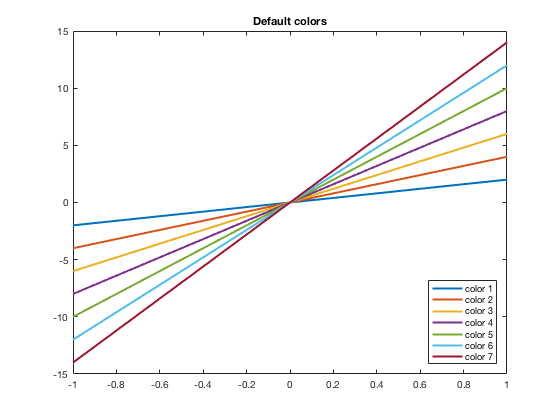Another thing that changed starting in the R2014b version is that the and automatically cycles through the colors. In the past, each new command would start with the first color (blue) and you would have to manually change the color. Now it will automatically move to the next color(s). See below for how to manually adjust the colors.

plot(x,2*x, 'LineWidth', 2) hold on plot(x,4*x, 'LineWidth', 2) plot(x,6*x, 'LineWidth', 2) plot(x,8*x, 'LineWidth', 2) plot(x,10*x, 'LineWidth', 2) plot(x,12*x, 'LineWidth', 2) plot(x,14*x, 'LineWidth', 2) hold off legend('color 1', 'color 2', 'color 3', 'color 4', 'color 5', 'color 6', 'color 7', 'Location', 'SouthEast') title('Hold On Now Cycles Through Colors')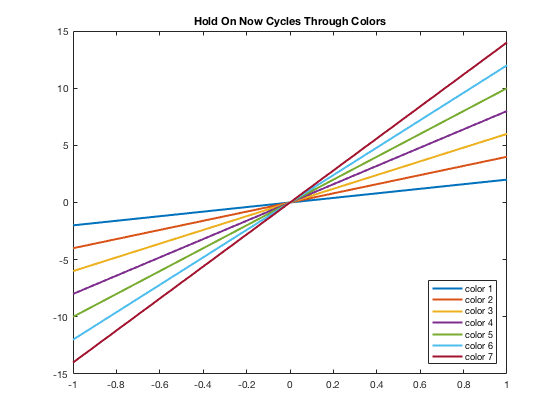Default Colors in 3D Graphs

If using , to change the look of it you would want to change . Note that the name of this colormap is "parula" while previous to R2014b, it was "jet"

[x,y]=meshgrid(linspace(0,10)); z=sin(x).*cos(y); mesh(x,y,z) title('Default colors for mesh')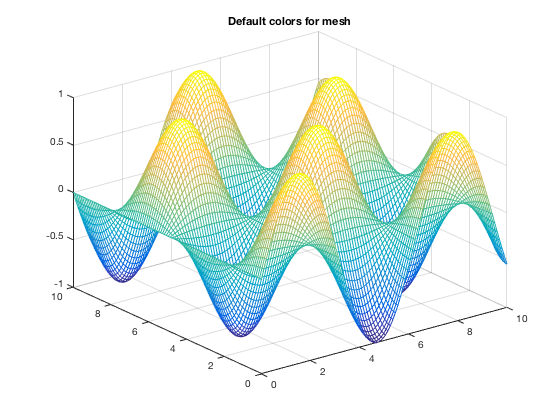[x,y]=meshgrid(linspace(0,10)); z=sin(x).*cos(y); mesh(x,y,z) colormap(jet) title('Default colors for mesh BEFORE 2014b')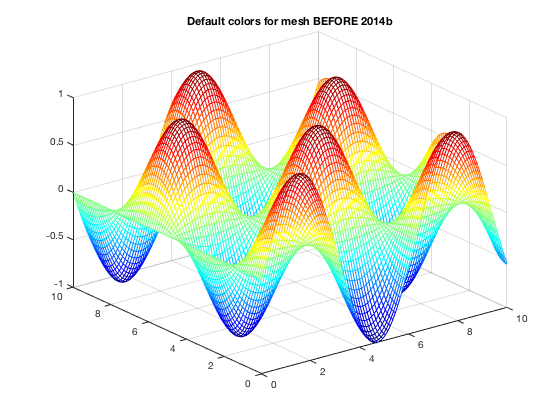Using Basic Colors in Graphs

The eight basic colors are known by either their short name or long name (RGB triplets are also included).

Long NameShort NameRGB Triplet
blueb[0,0,1]
blackk[0,0,0]
redr[1,0,0]
greeng[0,1,0]
yellowy[1,1,0]
cyanc[0,1,1]
magentam[1,0,1]
whitew[1,1,1]

Example of how to change the color using short names is below. You can easily do the same thing using the long names.

x=linspace(-1,1); plot(x,2*x,'b') hold on plot(x,4*x,'k') plot(x,6*x,'r') plot(x,8*x,'g') plot(x,10*x,'y') plot(x,12*x,'c') plot(x,14*x,'m') hold off legend('b = blue (default)', 'k = black', 'r = red', 'g = green', 'y = yellow', 'c = cyan', 'm = magenta', 'Location', 'SouthEast') title('Eight Basic colors (w = white not drawn)')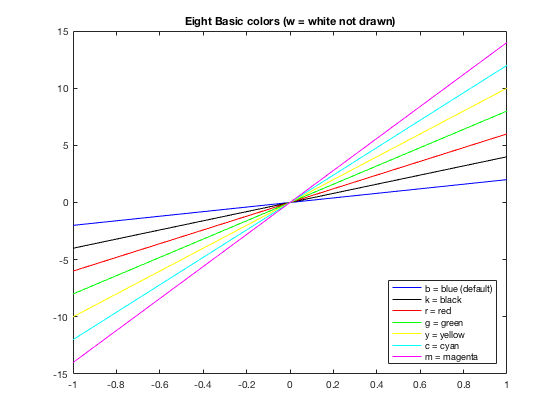Changing Colors

Many times you want to have more control of what colors are used. For example, I may want some data points drawn in the same color as the curve. Or I have a piece-wise graph that I want to have all the same color. There are several ways to do this. One is to use the default colors and "resetting" the order, which is shown here. Others involve using the RGB triplet (see next section).

x=linspace(-10,10); y=3*sin(x); xp=[0:pi/2:10]; yp=3*sin(xp); x1 = linspace(-10,-0.1); x2=linspace(0.1,10); y1=1./x1; y2=1./x2; plot(x,y) hold on ax = gca; ax.ColorOrderIndex = 1; plot(xp,yp,'*') plot(x1,y1) ax.ColorOrderIndex = 2; plot(x2,y2) hold off axis equal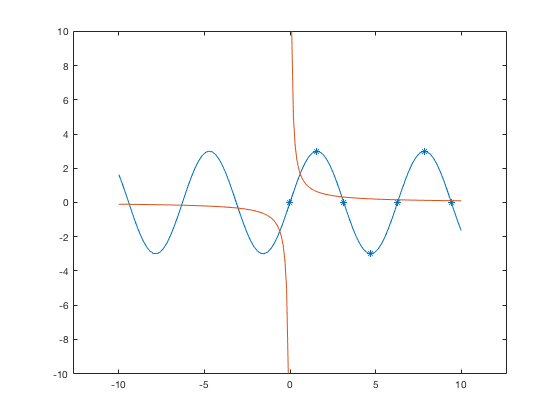As you may see, this could get confusing to keep track of. Thus it may be easier to use the RGB triplets, and even name them ahead of time. This is discussed in the section below.

Using RGB triplets to change colors

One can specify colors using a vector that gives the RGB triple where in MATLAB, each of the three values are numbers from 0 to 1. Usually RGB colors have values from 0 to 255. You can use those numbers and divide the vector by 255 to use within MATLAB. Thus knowing the MATLAB RGB triples for the colors can be useful. From the table above, we can define the default colors to work with them or can put in the RGB triplet (as a vector) directly into the command. Both are shown in this example.

color1=[0,0.4470, 0.7410]; t=linspace(0,10); t2=0:pi/2:10; plot(t,sin(t), 'LineWidth',2) hold on plot(t2,sin(t2),'s','MarkerEdge',color1,'MarkerFace',[0.9290,0.6940, 0.1250],'MarkerSize',9) hold off ylim([-1.5,1.5]) title('Using MATLAB RGB triplets in 2D graphs')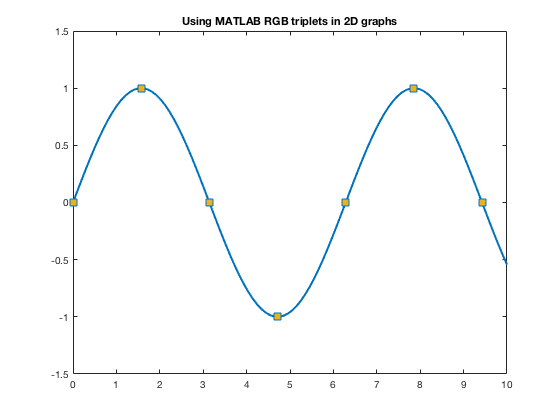For other colors, you can look up their RGB code on many websites such as RGB Color Codes Chart or HTML Color Picker to see the RGB codes (or hex codes, etc.) For example, at these RGB Color websites, you will be given R=255, G=0, B=0 for red. So you can use 1/255[255,0,0] to get the color of red to use as a color in MATLAB.

The official color for Loyola Green is given as RGB:0-104-87, and Loyola Gray is given as RGB:200-200-200 (found on Loyola's Logos/University Signature page. Here's how one can use those colors in MATLAB.

loyolagreen = 1/255*[0,104,87]; loyolagray = 1/255*[200,200,200];

Now one can use these colors to specify the color of markers, lines, edges, faces, etc.

t=linspace(0,10); t2=0:pi/2:10; plot(t,sin(t),'Color', loyolagreen, 'LineWidth',2) hold on plot(t2,sin(t2),'s','MarkerEdge','k','MarkerFace',loyolagray,'MarkerSize',9) hold off ylim([-1.5,1.5]) title('Using Other RGB colors in 2D graphs')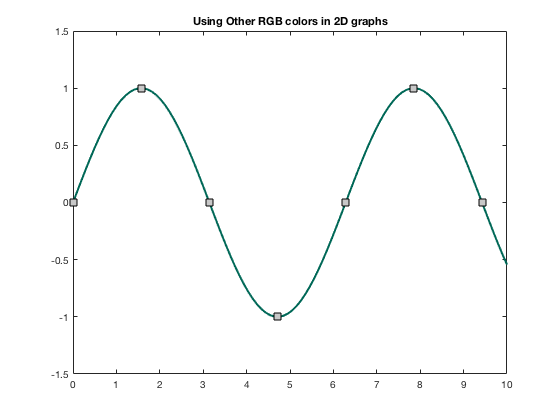Changing colors in 3D Graphs

If using , to change the look of it you can change to a different as discussed in https://www.mathworks.com/help/matlab/ref/colormap.html. This was done above when showing the previous default colormap. Here are some more.

Warning! Once you change the colormap, it will keep that colormap for all subsequent 3D plots within the same figure or MATLAB session until you use , or open a new window.

[x,y]=meshgrid(linspace(0,10)); z=5*sin(x).*cos(y); ax1=subplot(2,2,1); mesh(x,y,z) colormap(ax1,parula) title('Default colormap(parula)') ax2=subplot(2,2,2); mesh(x,y,z) colormap(ax2,jet) title('Colormap(jet)') ax3=subplot(2,2,3); colormap(ax3,bone) mesh(x,y,z) title('Colormap(bone)') ax4=subplot(2,2,4); colormap(ax4,summer) mesh(x,y,z) title('Colormap(summer)')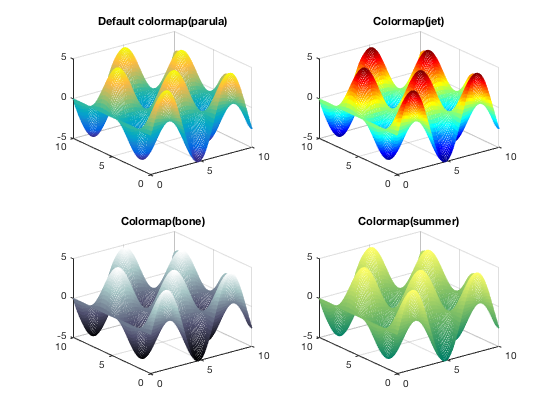For and , you can change and/or to be uniform, rather than using colormaps.

close all mesh(x,y,z,'EdgeColor',color1) title('Changing EdgeColor')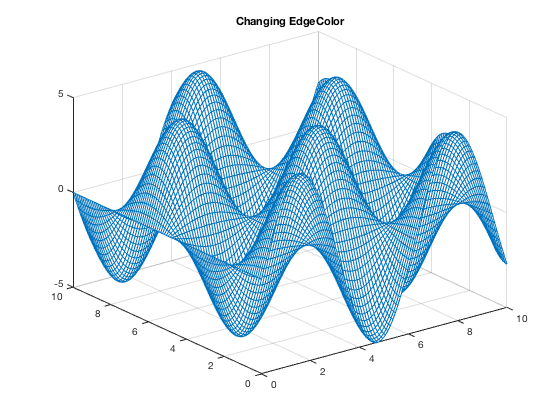Note that you can get as fancy as you want with your own coloring schemes. These are discussed in MATLAB's documentation (and elsewhere!) but I am not discussing here.surf(x,y,z) title('Default colors for surf')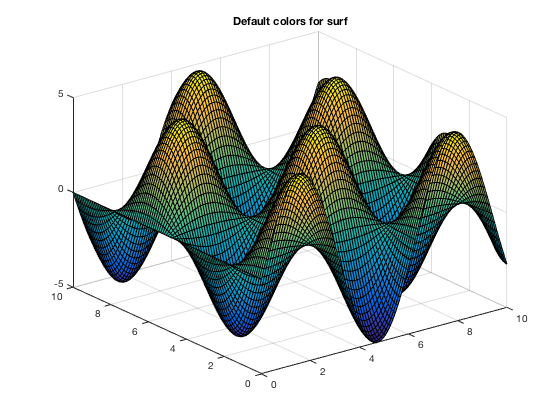surf(x,y,z,'EdgeColor', 'y', 'FaceColor','r') title('Changing EdgeColor and FaceColor using surf')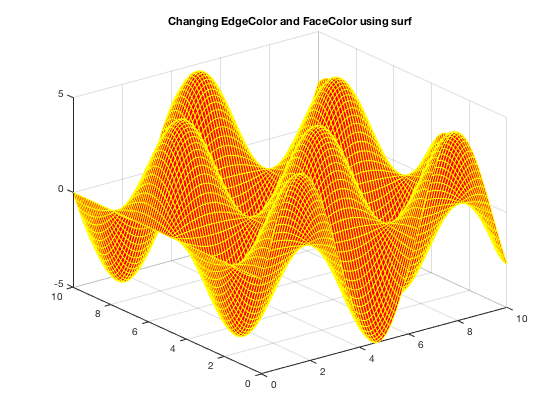surf(x,y,z,'EdgeColor',loyolagreen,'FaceColor',loyolagray) title('Using RGB colors in 3D graphs')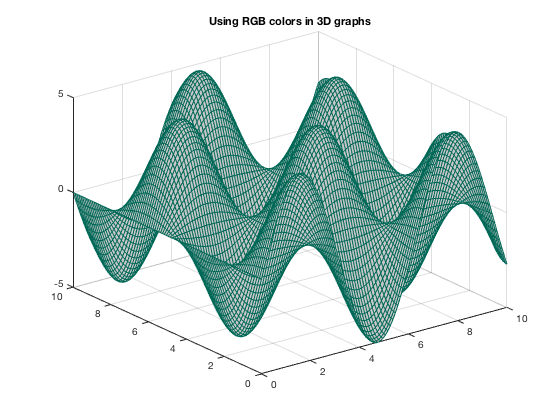BACK TO MAIN PLOTTING PAGE

Sours: http://math.loyola.edu/~loberbro/matlab/html/colorsInMatlab.html

5225 5226 5227 5228 5229